## RS Aggarwal Class 7 Solutions Chapter 4 Rational Numbers CCE Test Paper

These Solutions are part of RS Aggarwal Solutions Class 7. Here we have given RS Aggarwal Solutions Class 7 Chapter 4 Rational Numbers CCE Test Paper.

Other Exercises

Question 1.
Solution: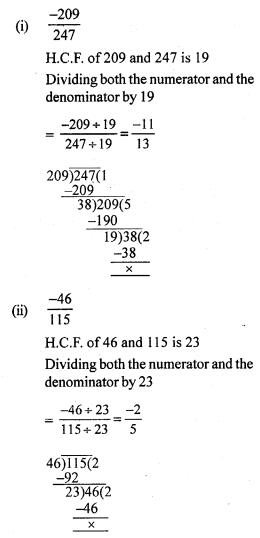Question 2.
Solution: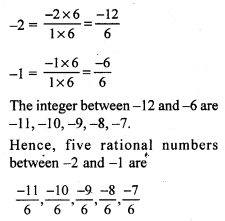Question 3.
Solution:
Let the required number be x.Question 4.
Solution:
Let the other number be x.Question 5.
Solution: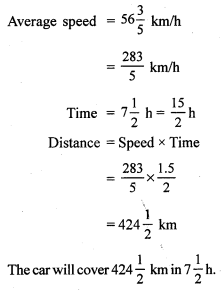Question 6.
Solution:
Let the required number be x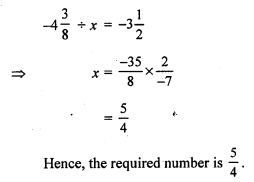Question 7.
Solution:
Length of rope = 45 m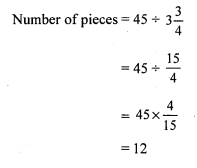Question 8.
Solution: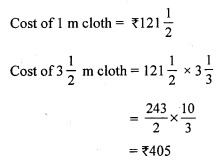Mark (✓) against the correct answer in each of the following :
Question 9.
Solution: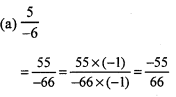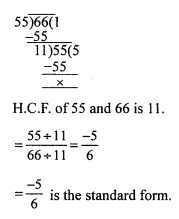Question 10.
Solution:Question 11.
Solution:Question 12.
Solution:Question 13.
Solution: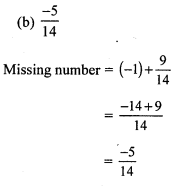Question 14.
Solution:Question 15.
Solution: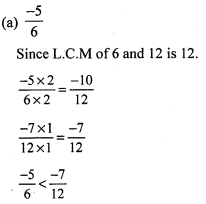Question 16.
Solution: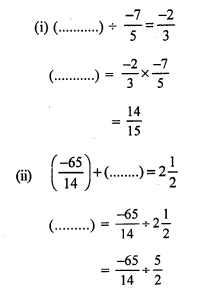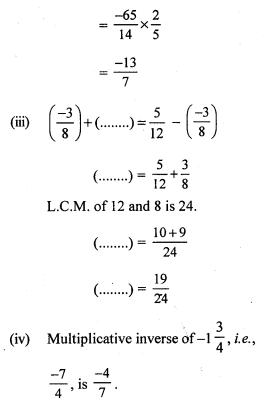Question 17.
Solution:
(i) False.
This is because $$\frac { -15 }{ -11 }$$ = $$\frac { 15 }{ 11 }$$, which lies to the right of 0.
(ii) True
(iii) True
(iv) False
L.C.M. of 5 and 3 is 15.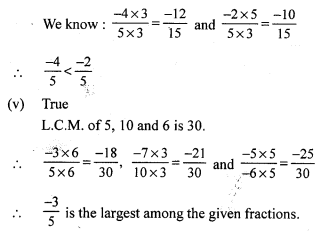Hope given RS Aggarwal Solutions Class 7 Chapter 4 Rational Numbers CCE Test Paper  are helpful to complete your math homework.

If you have any doubts, please comment below. Learn Insta try to provide online math tutoring for you.